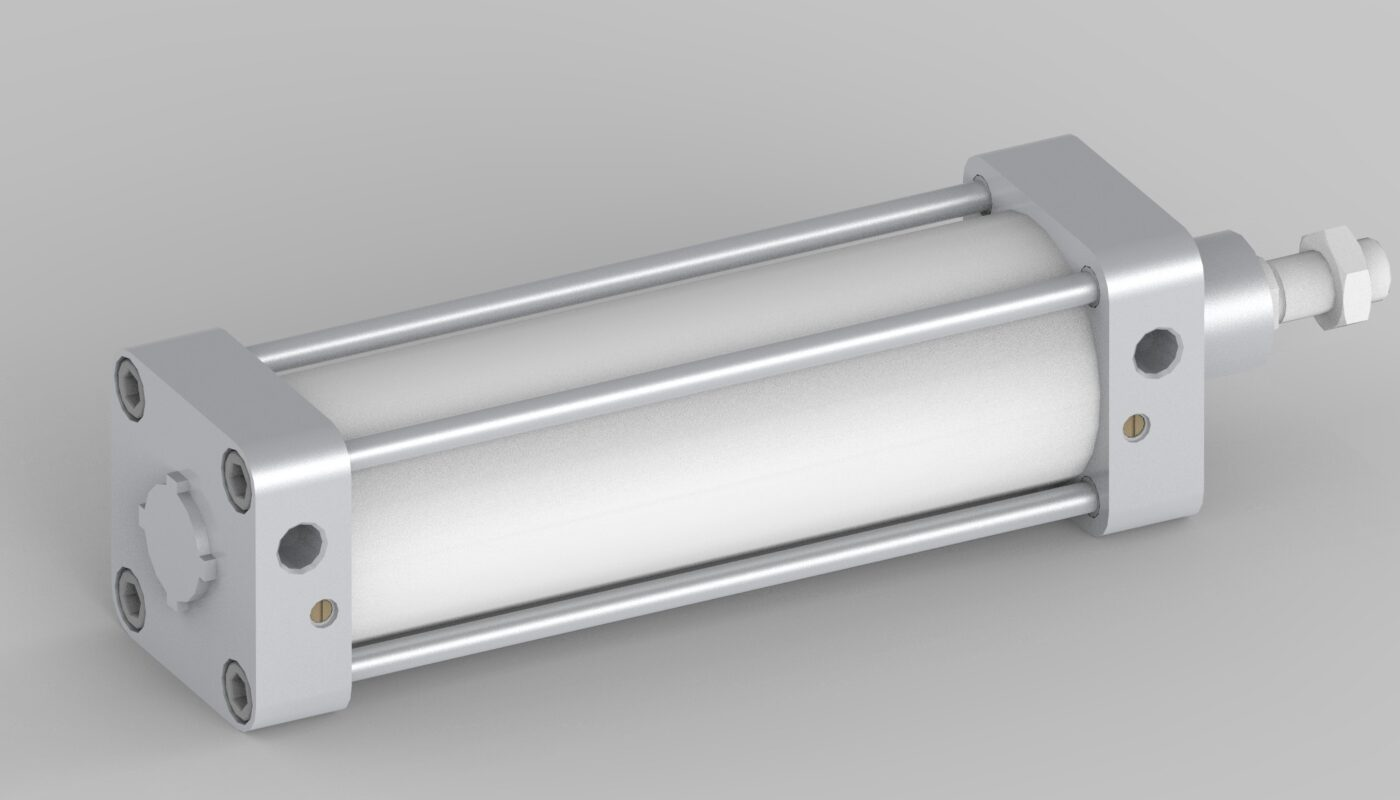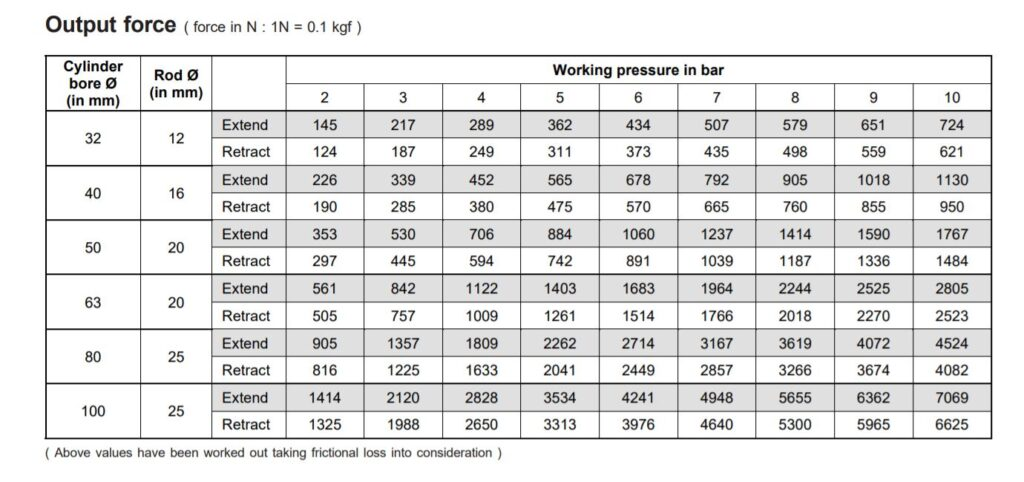December 4, 2023# Pneumatic cylinder output force calculations | Pneumatic cylinder selection guide

Pneumatic cylinder force calculations formulas includes force calculation, air consumption, Output power. Pneumatic systems use compressed air to move object using cylinder, motor or other mechanical parts. Hydraulic and Pneumatic systems have wide variety of applications in the industry includes automobile, packaging, medical and construction. All pneumatic system used compressed air to move and operate actuators. Pneumatic systems having no of components like Actuators, Compressors, Regulators, Pressure gauges, Check valves, Buffer tank, Filter Regulation and Lubrication unit (FRL), Pressure relief valves or Safety valve, Pneumatic manifolds, Pneumatic tubes and various pneumatic valves.

We will calculate right cylinder diameter for your applications by doing force calculations. As well as air consumption in cylinder.

## Packing friction (Fr)

The minimum amount of force which is required to move the piston in cylinder under no load condition is called packing friction.

In case of cylinders the piston is rigidly in the cylinder. Due to rubber piston seal and tight fittings, it requires some amount of force just to move the piston.

Generally, packing friction is considered to be same for both extension and retraction. That is same amount of force is required to pull as well as to push the piston in the cylinder under no load.

##### Net force required = Working load + Packing friction

Were,

D = Diameter of piston or bore diameter of cylinder in meter.

d= Diameter of piston rod in meter.

F= Force in Newton

V= Velocity in m/s

Q= Discharge in m^3/s

P=Power in watts

P= Pressure in N/m^3

A= Effective area on which the pressure acts is A during extension and (A-a) during retraction.

Were,

• Area of piston (A)
• Area of piston rod (a)

## During extension of cylinder.

1. Force (F)= Pressure (N/m^2) X Effective area (m^2)
2. Velocity (V)= Rate of flow (m^3/s) / Effective area (m^2) V=Q/A
3. Power Input to cylinder (P in) =Pressure X Rate of flow
4. Power Output of cylinder (P out) = Force X velocity of piston

If packing friction is not considered then effective input power is equal to effective output and efficiency of cylinder is 100%.

If packing friction is considered then effective input power is not equal to output power and efficiency is equal to effective power output divide by effective input power.

(P out) eff= (F net) X V

Force net = P X A – 10% of F net

Considering packing friction force is 10 percent of total force.

F net = P X A – 0.1 F net

Force net = P X A

F net = P X A÷1.1

Air consumption to cylinder calculations

Q = Area X Stroke X Compression ratio factor

Q = Area X Stroke X (P atm + P ÷P atm)

Note: In return or retraction stroke Area is (A-a)

#### 1. To select pneumatic cylinder for your application.

Example 1,

Let us consider here mass = 100 kg,

Working pressure of air is 12 bar.

F = 100 x 9 .81

= 981 N

Putting in force calculation formula,

F = Pressure (N/mm^2) x Area (mm^2)

981 = 1.2 x (3.14/4 x D2)

D2= 1041.4012

D = 32.27 mm

And considering factor of safety, 1.5

D = 32.27 x 1.5

= 48.40 mm

48.40 mm diameter is not available.

D= 50 mm.

#### 2. Calculating output force of cylinder.

Let us consider here cylinder bore or piston diameter is 63 mm

Working pressure is 6 bar

63 bore diameter cylinder is right choice or not?

Pushing load  =m x g x µ

=500*9.81*0.3

=1471.5 N

Cylinder output force = Pressure (N/mm^2) X Area (mm^2)

= 0.6 x (3.14/4 x 632)

F=1870 N

Above value is without considering packing friction,

packing friction force is 10 percent of cylinder output force

=0.1 X 1870

=187 N

Net force = 1870-187

F out =1683 N

We have Janatics catalogue for cylinder output force with considering packing friction.

For cylinder diameter 63 mm and working pressure 6 bar cylinder output force is 1683 N, so selected cylinder for your application is perfect.#### SKY HIGH eLearn

View all posts by SKY HIGH eLearn →

## 2 thoughts on “Pneumatic cylinder output force calculations | Pneumatic cylinder selection guide”

1.A KARTHIKEYAN says:

For easily & quickly understand

2.NICOLAAS. LOUW says:

PLEASE ADVISE ME WHAT KIND OF CILINDER I MUST CHOOSE FOR SPLITTING HARD WOOD ?? HYDRAULIC OR AIR CILINDER ?? I WANT A FAST ACTING CILINDER . HOW MANY TONS FORCE WILL BE SUFFICIENT ?? WHICH CILINDER WILL YOU RECOMMEND .
THANK YOU .